Next: Yielding of an elastic Up: Perihelion precession of Mercury Previous: Evaluation of disturbing function

# Secular perihelion precession rate

According to Equations (B.23), (B.38), and (B.39), Mercury's instantaneous perihelion precession rate, due to the perturbing influence of some other planet in the solar system, takes the form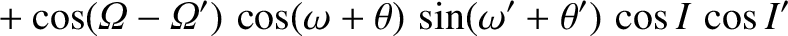(B.39)

where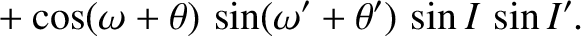(B.40)

However, we are only interested in the secular precession rate; that is, the mean rate over some timescale that is much longer than either the orbital period of Mercury or that of the perturbing planet. We can obtain a formula for the secular precession rate by averaging the previous expression over the orbits of the two planets. In other words,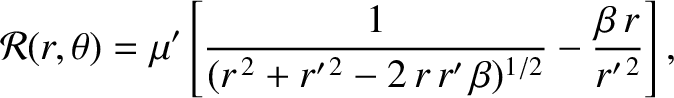(B.41)

where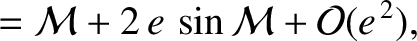and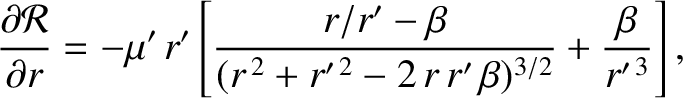are the mean anomalies of Mercury and the perturbing planet, respectively. (See Section 4.11.) Note that we must average in terms of the mean anomalies, rather than the true anomalies, because the former increase uniformly in time (unlike the latter). In fact,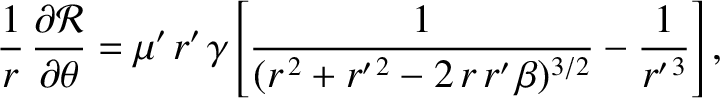and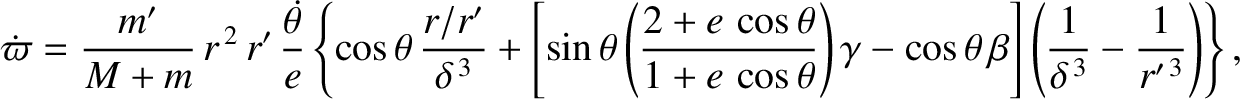, where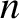and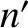are the mean orbital angular velocities of Mercury and the perturbing planet, respectively. (See Section 4.11.) Furthermore,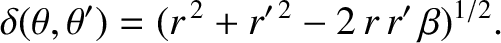is a constant of the perturbing planet's motion. (See Section 4.8.) Hence,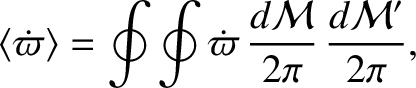. Thus, we obtain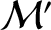where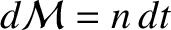,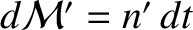,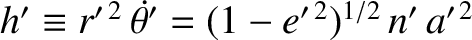,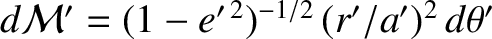, and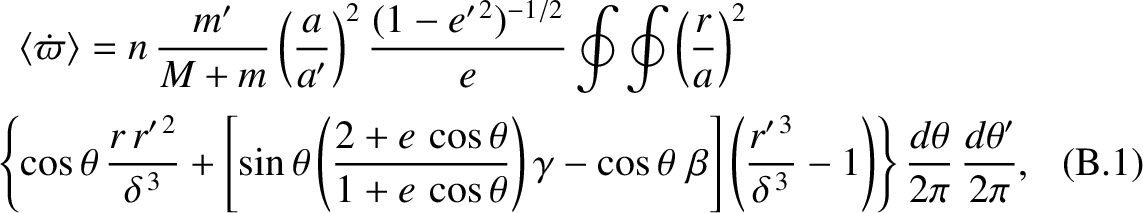are specified in Equations (B.31), (B.32), (B.35), (B.36), and (B.41) respectively. Here,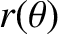arc seconds per year (whereis measured in astronomical units). The previous expression can be simplified somewhat, because some terms are obviously annihilated by the integration in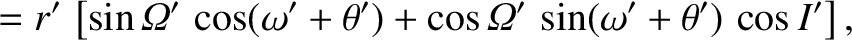. In fact,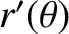Table B.1: Contributions to the secular precession rate of Mercury's perihelion from the other planets in the solar system.
 Planet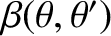Venus 2.7745 Earth 0.9084 Mars 0.0248 Jupiter 1.5400 Saturn 0.0731 Uranus 0.0014 Neptune 0.0004 Total 5.3226

Table B.1 shows the contributions to the secular precession rate of Mercury's perihelion, due to the perturbing influence of the other planets in the solar system, calculated from Equation (B.44), using the planetary mass and orbital element data given in Table 4.1. It can be seen that the dominant contributions to the precession rate come from Venus, Earth, and Jupiter. Furthermore, the total precession rate is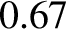arc seconds per year.Next: Yielding of an elastic Up: Perihelion precession of Mercury Previous: Evaluation of disturbing function
Richard Fitzpatrick 2016-03-31